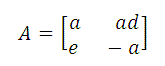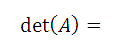Free Compass Math Test Practice Questions with Answers - Sample 4

 A set of college algebra and trigonometry questions, with answers, similar to the questions in the compass math test are presented. The answers to the suggested questions are at the bottom of the page and the solutions with full explanations to these questions are also included. If f(x) = 2x - 3, g(x) = -2x3 + 2 and h(x) = 3x, then h(2) + f(g(-1)) = A) 10 B) -14 C) 11 D) 5 E) 1 If 3x2 / 34x = 1/81, then x = A) -1 B) 0 C) 1 D) 2 E) 3 What are the solutions of x(x - 2) = 5? A) 2 and 7 B) 5 and 2 C) -5 and 7 D) -1 + sqrt(6) and -1 - sqrt(6) E) 1 - sqrt(6) and 1 + sqrt(6) The formula for the nth term, an, of an arirhmetic progression is given by an = a1 + (n - 1)d, where a1 represents the first term of the progession and d represents its common difference. What is the value of the 20th term of the arithmetic progression 4, 7, 10,...? A) 61 B) 64 C) 58 D) 67 E) 20 In a a 16-by-12 rectangle, what is the perimeter of the triangle formed by two sides of the rectangle and the diagonal? A) 56 B) 48 C) 192 D) 28 E) 40 If sqrt(-1) = i, then (-2 + 2 i)5 = A) -32 + 32 i B) 32 - 32 i C) -32 - 32 i D) 128 - 128 i E) -128 + 128 i For all values of x > 0, log[( x2 cube root(7) ] = A) log [ x2 + cube root(7) ] B) 2log(x) + (1/3)log(7) C) log(x) + log(7) D) x2 + cube root(7) E) (7/3)log(x) Which of these interval represents all the real values that are the range of y = 1 / (4 - x2) A) (-infinity , 0)U[1/4 , +infinity) B) (1/4 , +infinity) C) (-infinity , 0)U(1/4 , +infinity) D) [1/4 , +infinity) E) (-infinity , + infinity) Which of these intervals represents all values of x that makes sqrt(x2 + 2x) a real number? A) (-infinity , -2) U [0 , +infinity) B) (-infinity , -2] U [0 , +infinity) C) (-infinity , -2] U (0 , +infinity) D)(-infinity , -2) U (0 , +infinity) E) (-infinity , +infinity) log 32 + log 16 = A) log 48 B) 3log 16 C) 9 log 2 D) 12 log 2 E) 8 log 2 If 9(x + 1) = 3 * 9y, then A) x = y + 1 B) x + 1 = y C) x = y D) 2x = 2y - 1 E) x = y + 1 If A is a matrix given by, thenA) a(a + ed) B) -a(a - ed) C) a + ed D) -a(-a + ed) E) -a(a + ed) If cos(80o) = a and cos(60o)cos(20o) = b, then sin(60o)sin(20o) = A) a + b B) a - b C) a b D) a/b E) b - a Which pair of functions have the same graph? A) sin(x) and cos(x + 3 Pi/2) B) sin(x) and cos(x + Pi/2) C) sin(x) and cos(x + Pi) D) sin(x) and cos(x - 3 Pi/2) E) sin(x) and cos(x + 5 Pi/2) Which of these functions have the highest period? A) cos(x + 3 Pi/2) B) sin(2x - Pi) C) cos(0.2x) D) 10 sin(x) E) cos(200x) What is the measure of x, 0 < x < 5 Pi / 2, if |- sin(x) + 1| = 2 A) 2 Pi B) 3 Pi / 2 C) Pi / 2 D) Pi E) 2 Pi / 3 If Pi < x < 2 Pi and cos(x) = - 1 / 2, then x = A) Pi / 3 B) 3 Pi / 2 C) 5 Pi / 3 D) 4 Pi / 3 E) 5 Pi / 4 [ cos(t) / cot(t) ] csc2(t) = A) sin(t) B) csc(t) C) cos(t) D) tan(t) E) cot(t) If f(u) = 2 cos(u) + 5 and g(v) = 0.5 sin(2v), what is f(g(Pi / 2)) A) 7 B) 0 C) 5 D) 3 E) -3 If Pi < x < 2 Pi and tan(x) = 1/4, then sin(x) = A) 1 B) 1 / sqrt(17) C) 1 / sqrt(15) D) - 1 / sqrt(17) E) - 1 / sqrt(15)